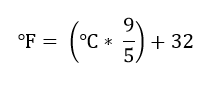## C program to convert temperature from degree celsius to fahrenheit

Write a C program to input temperature in Centigrade and convert to Fahrenheit. How to convert temperature from degree centigrade to degree Fahrenheit in C programming. Logic to convert temperature from Celsius to Fahrenheit in C.

#### Required knowledge

Arithmetic operators, Variables and expressions, Data types, Basic input/output

#### Temperature conversion formula

Temperature conversion formula from degree Celsius to Fahrenheit is given by -

#### Celsius to Fahrenheit conversion formula#### Logic to convert temperature from Celsius to Fahrenheit

The logic of temperature conversion exists in converting mathematical formula to C expression. You just need to convert mathematical formula of temperature in C language. Rest is simple input/output.

Below is the step by step descriptive logic to convert temperature from degree Celsius to Fahrenheit.

Input temperature in Celsius from user. Store it in some variable say celsius.

Apply formula to convert the temperature to Fahrenheit i.e. fahrenheit = (celsius * 9 / 5) + 32.

Print the value of fahrenheit.

Read more - Program to convert Fahrenheit to Celsius

#### Program to convert temperature from Celsius to Fahrenheit

```
/**
* C program to convert temperature from degree celsius to fahrenheit
*/

#include <stdio.h>

int main()
{
float celsius, fahrenheit;

/* Input temperature in celsius */
printf("Enter temperature in Celsius: ");
scanf("%f", &celsius);

/* celsius to fahrenheit conversion formula */
fahrenheit = (celsius * 9 / 5) + 32;

printf("%.2f Celsius = %.2f Fahrenheit", celsius, fahrenheit);

return 0;
}
```

%.2f is used to print fractional numbers up to two decimal places. You can also use %f to print fractional normally up to six decimal places.

##### Output:
```
Enter temperature in Celsius: 100
100 Celsius = 212.00 Fahrenheit
```
```
Recommended posts

Loop programming exercises index.
Basic programming exercises index.
If else programming exercises index.
Array programming exercises index.
String programming exercises index.
Pointer programming exercises index.
Star Patterns exercises index.
Number Patterns exercises index.
alphabet Patterns exercises index.
series program exercises index.

```